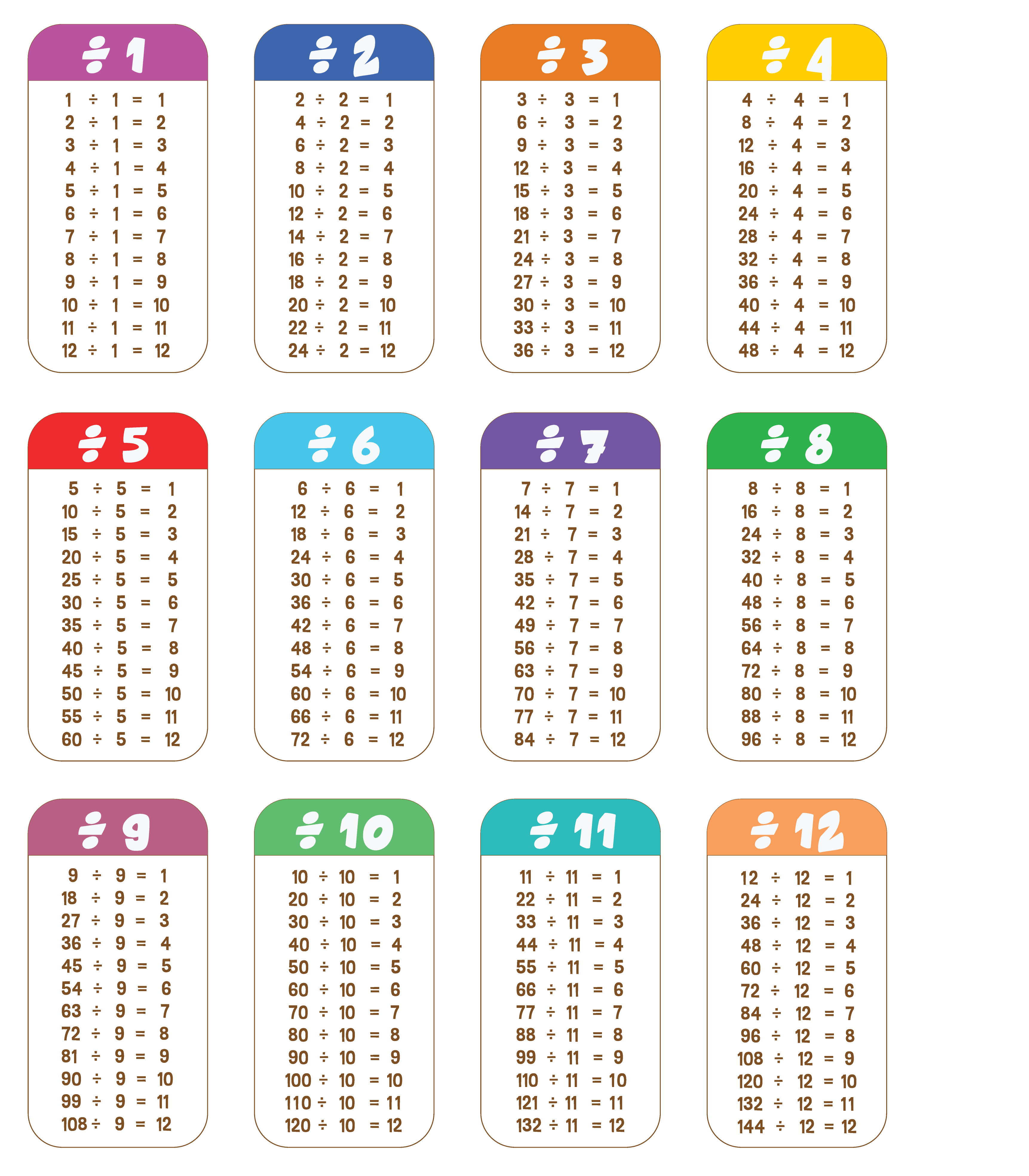Let's look at number sentences and work out if they're true or false.

Remember, a number sentence is true when both sides are equal.

Question

Are these number sentences true or false?

15 ÷ 3 = 5

The sentence is true. Both sides of the sentence are equal.

16 ÷ 4 = 5

The sentence is false. 16 ÷ 4 = 4.

24 ÷ 8 = 2

The sentence is false. 24 ÷ 8 = 3

Have a look at the tables below and when you’re ready, let's begin.